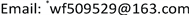﻿ 广义GrO¨tzsch环函数的精确界及应用 Sharp Inequalities and Application of the Generalized GrO¨tzsch Ring Function

Pure Mathematics
Vol. 09  No. 03 ( 2019 ), Article ID: 30067 , 5 pages
10.12677/PM.2019.93032

Sharp Inequalities and Application of the Generalized Grötzsch Ring Function

Fei Wang1*, Peigui Zhou2, Xiaoyu Wang1

1Teaching Section of Mathematics, Zhejiang Institute of Mechanical and Electrical Engineering, Hangzhou Zhejiang

2Keyi College of Zhejiang Sci-Tech University, Shangyu ZhejiangReceived: Apr. 9th, 2019; accepted: Apr. 20th, 2019; published: May 5th, 2019ABSTRACT

In this paper, we study some monotonicity properties of certain functions defined in term of generalized Grötzsch ring function and some elementary functions, and get new sharp inequalities. Furthermore, we also obtain the lower bound of generalized Hersch-Pfluger distortion function by applying these results in Ramanujan modular equation theory.

Keywords:Generalized Grötzsch Ring Function, Sharp Inequality, Ramanujan Modular Equations, Generalized Hersch-Pfluger Distortion Function

1浙江机电职业技术学院数学教研室，浙江 杭州

2浙江理工大学科技与艺术学院，浙江 上虞Copyright © 2019 by author(s) and Hans Publishers Inc.

This work is licensed under the Creative Commons Attribution International License (CC BY).

http://creativecommons.org/licenses/by/4.0/1. 引言

$\Gamma \left(x\right)={\int }_{0}^{\infty }{t}^{x-1}{\text{e}}^{-t}\text{d}t,\text{\hspace{0.17em}}\text{\hspace{0.17em}}B\left(x,y\right)=\frac{\Gamma \left(x\right)\Gamma \left(y\right)}{\Gamma \left(x+y\right)},\text{\hspace{0.17em}}\text{\hspace{0.17em}}\psi \left(x\right)=\frac{{\Gamma }^{\prime }\left(x\right)}{\Gamma \left(x\right)}$ (1)

$\gamma =\underset{n\to \infty }{\mathrm{lim}}\left[\underset{k=1}{\overset{n}{\sum }}\frac{1}{k}-\mathrm{log}n\right]=\text{0}\text{.57721566}\cdots$，是Euler-Mascheroni常数，则

$\psi \left(1\right)=-\gamma$ , $\psi \left(1/2\right)=-\gamma -\mathrm{log}4$ (2)

$\left(0,\infty \right)×\left(0,\infty \right)$ 上定义Ramanujan常数   为：

$R\left(a,b\right)=-\psi \left(a\right)-\psi \left(b\right)-2\gamma$ (3)

$b=1-a$ 时，式(3)记为

$R\left(a\right)=R\left(a,1-a\right)=-\psi \left(a\right)-\psi \left(1-a\right)-2\gamma$ ,

$F\left(a,b;c;z\right)={}_{2}F{}_{1}\left(a,b;c;z\right)=\underset{n=0}{\overset{\infty }{\sum }}\frac{\left(a,n\right)\left(b,n\right)}{\left(c,n\right)}\frac{{z}^{n}}{n!}$ , $|z|<1$ (4)

$a\in \left(0,1\right)$，第一类、第二类广义椭圆积分分别定义  为：

${K}_{a}={K}_{a}\left(r\right)=\frac{\text{π}}{2}F\left(a,1-a;1;{r}^{2}\right),\text{\hspace{0.17em}}\text{\hspace{0.17em}}{{K}^{\prime }}_{a}={{K}^{\prime }}_{a}\left(r\right)={K}_{a}\left({r}^{\prime }\right)$ (5)

${E}_{a}={E}_{a}\left(r\right)=\frac{\text{π}}{2}F\left(a-1,1-a;1;{r}^{2}\right),\text{\hspace{0.17em}}\text{\hspace{0.17em}}{{E}^{\prime }}_{a}={{E}^{\prime }}_{a}\left(r\right)={E}_{a}\left({r}^{\prime }\right)$ (6)

${\mu }_{a}\left(r\right)=\frac{\text{π}}{2\mathrm{sin}\left(a\text{π}\right)}\frac{{{K}^{\prime }}_{a}\left(r\right)}{{K}_{a}\left(r\right)}$ (7)

$\frac{F\left(a,1-a;1;1-{s}^{2}\right)}{F\left(a,1-a;1;{s}^{2}\right)}=p\frac{F\left(a,1-a;1;1-{r}^{2}\right)}{F\left(a,1-a;1;{r}^{2}\right)}$ (8)

${\mu }_{a}\left(s\right)=p{\mu }_{a}\left(r\right),p>0$ (9)

$s={\phi }_{K}\left(a,r\right)={\mu }_{a}^{-1}\left({\mu }_{a}\left(r\right)/K\right)$ , $K=1/p$ (10)

$\mu \left(r\right)+{C}_{1}\left(1-{r}^{2}\right)\le {\mu }_{a}\left(r\right)\le \mu \left(r\right)+{C}_{1}-\left(a-1/2\right){r}^{2}$

$a=1/2$ 时等号成立，其中 ${C}_{1}=\left[R\left(a\right)-\mathrm{log}16\right]/2$

${\phi }_{1/K}\left(a,r\right)>{r}^{K}\mathrm{exp}\left\{\left(1-K\right)c\left(r\right)\right\}$ (11)

2. 引理

$\frac{\text{d}{K}_{a}}{\text{d}r}=2\left(1-a\right)\frac{{E}_{a}-{{r}^{\prime }}^{2}{K}_{a}}{r{{r}^{\prime }}^{2}}$ , $\frac{\text{d}{E}_{a}}{\text{d}r}=2\left(1-a\right)\frac{{E}_{a}-{K}_{a}}{r}$ (12)

$\frac{\text{d}{\mu }_{a}\left(r\right)}{\text{d}r}=-\frac{{\text{π}}^{2}}{4r{{r}^{\prime }}^{2}{K}_{a}^{2}}$ (13)

$F\left(x\right)=\frac{f\left(x\right)-f\left(a\right)}{g\left(x\right)-g\left(a\right)}$

$G\left(x\right)=\frac{f\left(x\right)-f\left(b\right)}{g\left(x\right)-g\left(b\right)}$

(1) $\frac{{\text{π}}^{2}/4-{r}^{\prime }{K}_{a}^{2}\left(r\right)}{{r}^{2}}$$\left(0,1\right)$$\left({\text{π}}^{2}\left[{a}^{2}+{\left(1-a\right)}^{2}\right]/4,{\text{π}}^{2}/4\right)$ 上严格单调递增。

(2) ${r}^{\prime }{K}_{a}\left(r\right)$$\left(0,1\right)$$\left(0,\text{π}/2\right)$ 上严格单调递减。

3. 主要结果及证明

$F\left(r\right)=\frac{{C}_{1}-\mathrm{exp}\left[{\mu }_{a}\left(r\right)-arth\left({r}^{\prime }\right)\right]}{{r}^{2}}$

$\left(0,1\right)$$\left({\left(1/2-a\right)}^{2}{C}_{1},{C}_{1}-1\right)$ 上严格单调递增。特别地，当 $0 时，

$\mathrm{log}\left[{C}_{1}-\left({C}_{1}-1\right){r}^{2}\right]+\mathrm{log}\left(1+{r}^{\prime }\right)<{\mu }_{a}\left(r\right)+\mathrm{log}r<\mathrm{log}{C}_{1}+\mathrm{log}\left[1-{\left(1/2-a\right)}^{2}{r}^{2}\right]+\mathrm{log}\left(1+{r}^{\prime }\right)$ (14)

$\begin{array}{c}\frac{{{f}^{\prime }}_{1}\left(r\right)}{{{f}^{\prime }}_{2}\left(r\right)}=\frac{1}{2}\frac{\mathrm{exp}\left[{\mu }_{a}\left(r\right)-arth\left({r}^{\prime }\right)\right]}{{r}^{\prime }}\frac{{\text{π}}^{2}/4-{r}^{\prime }{K}_{a}^{2}}{{r}^{2}}\frac{1}{{r}^{\prime }{K}_{a}^{2}}\\ =\frac{1}{2}\mathrm{exp}\left[{\mu }_{a}\left(r\right)-arth\left({r}^{\prime }\right)+\mathrm{log}\frac{1}{{r}^{\prime }}\right]\frac{{\text{π}}^{2}/4-{r}^{\prime }{K}_{a}^{2}}{{r}^{2}}\frac{1}{{r}^{\prime }{K}_{a}^{2}}\\ =\frac{1}{2}\mathrm{exp}\left[{\mu }_{a}\left(r\right)+\mathrm{log}\frac{r}{{r}^{\prime }}-\mathrm{log}\left(1+{r}^{\prime }\right)\right]\frac{{\text{π}}^{2}/4-{r}^{\prime }{K}_{a}^{2}}{{r}^{2}}\frac{1}{{r}^{\prime }{K}_{a}^{2}}\\ ={f}_{3}\left(r\right)\end{array}$

$\underset{r\to {0}^{+}}{\mathrm{lim}}F\left(r\right)=\underset{r\to {0}^{+}}{\mathrm{lim}}\frac{{{f}^{\prime }}_{1}\left(r\right)}{{{f}^{\prime }}_{2}\left(r\right)}={\left(1/2-a\right)}^{2}{\text{e}}^{\left(R\left(a\right)-\mathrm{log}4\right)/2}={\left(1/2-a\right)}^{2}{C}_{1}$ .

$G\left(r\right)=\frac{\mathrm{exp}\left[{C}_{2}-\left({\mu }_{a}\left(r\right)-\mu \left(r\right)\right)\right]-1}{{r}^{2}}$

$\left(0,1\right)$$\left(\alpha ,\beta \right)$ 上严格单调递增。特别地，当 $0 时，

$\frac{R\left(a\right)-\mathrm{log}16}{2}-\mathrm{log}\left(1+\alpha {r}^{2}\right)<{\mu }_{a}\left(r\right)-\mu \left(r\right)<\frac{R\left(a\right)-\mathrm{log}16}{2}-\mathrm{log}\left(1+\beta {r}^{2}\right)$ (15)

$\frac{{{g}^{\prime }}_{1}\left(r\right)}{{{g}^{\prime }}_{2}\left(r\right)}=\frac{{\text{π}}^{2}}{8}\mathrm{exp}\left\{{C}_{2}-\left[{\mu }_{a}\left(r\right)-\mu \left(r\right)\right]\right\}\frac{K+{K}_{a}}{{r}^{\prime }{K}^{2}}\frac{1}{{r}^{\prime }{K}_{a}^{2}}\frac{K-{K}_{a}}{{r}^{2}}={g}_{3}\left(r\right)$

$\underset{r\to {0}^{+}}{\mathrm{lim}}G\left(r\right)=\underset{r\to {0}^{+}}{\mathrm{lim}}\frac{{{g}^{\prime }}_{1}\left(r\right)}{{{g}^{\prime }}_{2}\left(r\right)}={\left(1/2-a\right)}^{2}=\alpha$ .

${\phi }_{1/K}\left(a,r\right)>{r}^{K}{\left\{{\text{e}}^{\left[R\left(a\right)-\mathrm{log}4\right]/2}\left[1-{\left(1/2-a\right)}^{2}{r}^{2}\right]\left(1+{r}^{\prime }\right)\right\}}^{1-K}$ (16)

${\phi }_{1/K}\left(r\right)>{r}^{K}{\left\{2\left(1+{r}^{\prime }\right)\right\}}^{1-K}$ (17)

Sharp Inequalities and Application of the Generalized GrO¨tzsch Ring Function[J]. 理论数学, 2019, 09(03): 254-258. https://doi.org/10.12677/PM.2019.93032

1. 1. Abramwitz, M. and Stegun, I.A. (1965) Handbook of Mathematical Functions with Formulas, Graphs and Mathematical Tables. Dover Publications, New York, 253-294.

2. 2. Qiu, S.L. and Vuorinen, M. (2005) Special Functions in Geometric Function Theory. In: Kühnau, R., Handbook of Complex Analysis: Geometric Function Theory, Elsevier, Amsterdam, 621-659.
https://doi.org/10.1016/S1874-5709(05)80018-6

3. 3. Qiu, S.L., Ma, X.Y. and Huang, T.R. (2017) Some Proper-ties of the Difference between the Ramanujan Constant and Beta Function. Journal of Mathematical Analysis and Ap-plications, 40, 114-129.
https://doi.org/10.1016/j.jmaa.2016.08.043

4. 4. Anderson, G.D., Vamanamurthy, M.K. and Vuorinen, M. (1997) Conformal Invariants, Inequalities, and Quasiconformal Maps. John Wiley & Sons, New York, 32-47.

5. 5. Anderson, G.D., Qiu, S.L., Vamanamurthy, M.K., et al. (2000) Generalized Elliptic Integrals and Modular Equations. Pacific Journal of Mathematic, 192, 1-37.
https://doi.org/10.2140/pjm.2000.192.1

6. 6. 裘松良. GrÖtzsch环与Ramanujan模方程[J]. 数学学报, 2000, 43(2): 283-290.

7. 7. Wang, G.D., Zhang, X.H. and Chu, Y.M. (2007) Ine-qualities for the Generalized Elliptic Integrals and Modular Functions. Journal of Mathematical Analysis and Applications, 331, 1275-1283.
https://doi.org/10.1016/j.jmaa.2006.09.070

8. 8. Alzer, H. and Richards, K. (2015) On the Modulus of the GrÖtzsch Ring. Journal of Mathematical Analysis and Applications, 432, 134-141.
https://doi.org/10.1016/j.jmaa.2015.06.057

9. 9. Zhang, X.H. (2017) On the Generalized Modulus. The Ramanu-jan Journal, 43, 405-413.

10. 10. Ma, X.Y., Chu, Y.M. and Wang, F. (2013) Monotonicity and Inequalities for the Gen-eralized Distortion Function. Acta Mathematica Scientia, 33, 1759-1766.
https://doi.org/10.1016/S0252-9602(13)60121-6

11. 11. 张孝惠, 王根娣, 褚玉明, 等. 广义偏差函数的单调性和不等式[J]. 数学年刊, 2007, 28(2): 183-190.

NOTES

*通讯作者。